# ISEE Upper Level Quantitative : How to find if triangles are similar

## Example Questions

### Example Question #1 : How To Find If Triangles Are Similar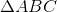and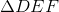are right triangles, with right angles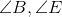, respectively.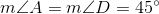and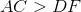.

Which is the greater quantity?

(a)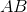(b)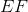It is impossible to tell from the information given.

(b) is greater.

(a) and (b) are equal.

(a) is greater.

(a) is greater.

Explanation:

Each right triangle is a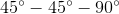triangle, making each triangle isosceles by the Converse of the Isosceles Triangle Theorem.

Since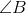and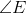are the right triangles, the legs are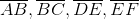, and the hypotenuses are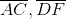.

By theTheorem,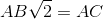and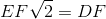., so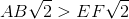and subsequently,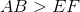.

### Example Question #2 : How To Find If Triangles Are Similar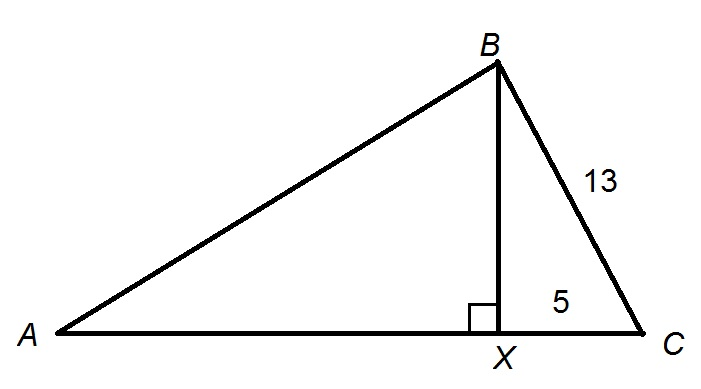Figure NOT drawn to scale.

In the above figure,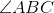is a right angle.

What is the ratio of the area of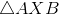to that of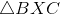?

144 to 25

12 to 5

13 to 5

169 to 25

144 to 25

Explanation:

The altitude of a right triangle from the vertex of its right angle divides the triangle into two smaller, similar triangles.

The similarity ratio oftocan be found by determining the ratio of one pair of corresponding sides; we will use the short leg of each,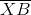and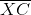.is also the long leg of, so its length can be found using the Pythagorean Theorem: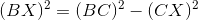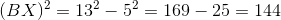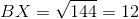The similarity ratio is therefore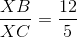.

The ratio of the areas is the square of this ratio: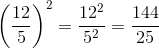- that is, 144 to 25.